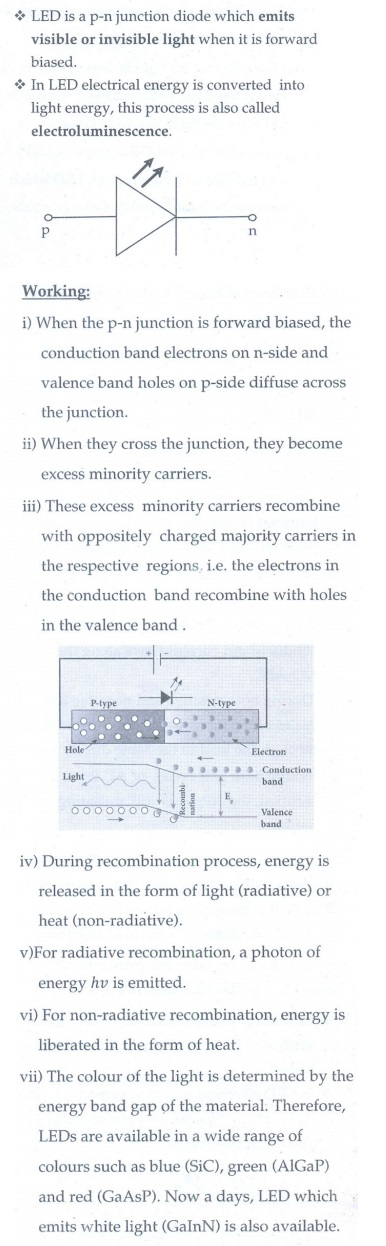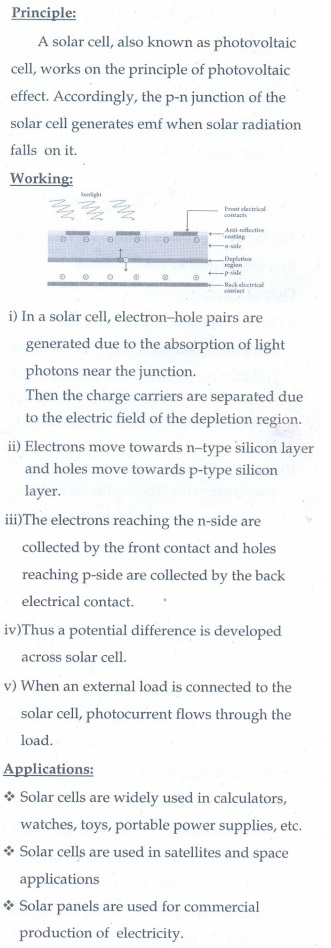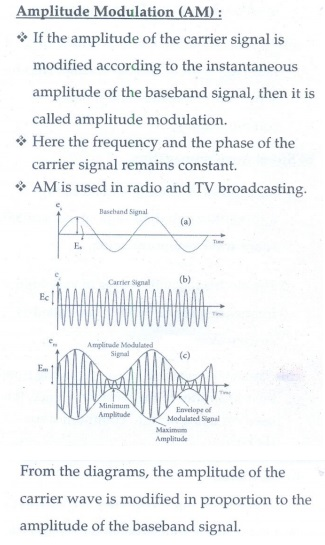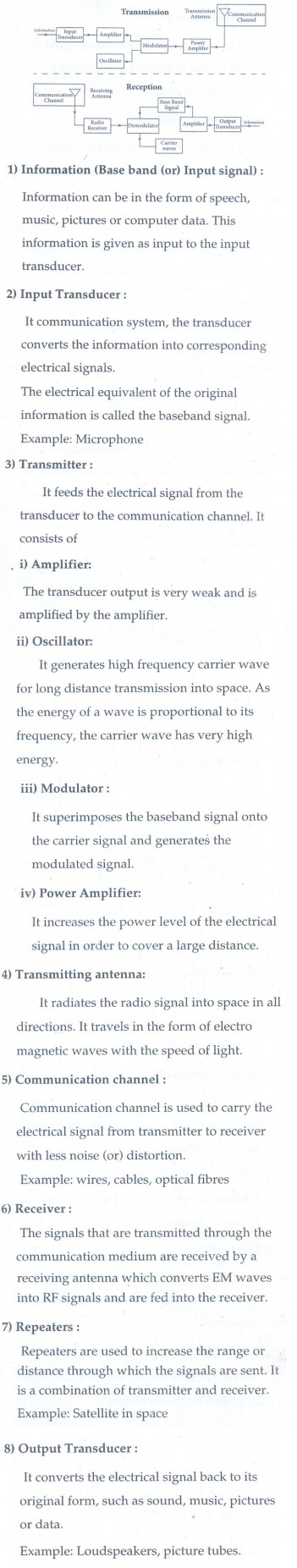Home | | Physics 12th Std | Long Answers

## Chapter: 12th Physics : UNIT 10b : Communication Systems

Physics : Communication Systems: Book Back Important Questions, Answers, Solutions: Long Answers

1. Elucidate the formation of a N-type and P-type semiconductors.2. Explain the formation of depletion region and barrier potential in PN junction diode.3. Draw the circuit diagram of a half wave rectifier and explain its working4. Explain the construction and working of a full wave rectifier.5. What is an LED? Give the principle of operation with a diagram.6. Write notes on Photodiode.7. Explain the working principle of a solar cell. Mention its applications.8. Sketch the static characteristics of a common emitter transistor and bring out the essential features of input and output characteristics.9. Transistor functions as a switch. Explain.10. Describe the function of a transistor as an amplifier with the neat circuit diagram. Sketch the input and output wave form.11. Give circuit symbol, logical operation, truth table, and Boolean of (i) AND gate (ii) OR gate (iii) NOT gate (iv) NAND gate (v) NOR gate and (vi) EX-OR gate12. State and prove De Morgan's First and Second theorems.13. Explain the amplitude modulation with necessary diagram.14. Explain the basic element of communication system with nessary block diagram.15. Explain the ground wave propagation and space wave propagation of electromagnetic wave through space.16. Fiber optic communication is gaining popularity among the various transmission media -justify.17. Mention the advantage and limitations of frequency modulation(FM).18. What is meant by satellite communication ? Give its applications.Tags : Communication Systems | Physics , 12th Physics : UNIT 10b : Communication Systems
Study Material, Lecturing Notes, Assignment, Reference, Wiki description explanation, brief detail
12th Physics : UNIT 10b : Communication Systems : Long Answers | Communication Systems | Physics